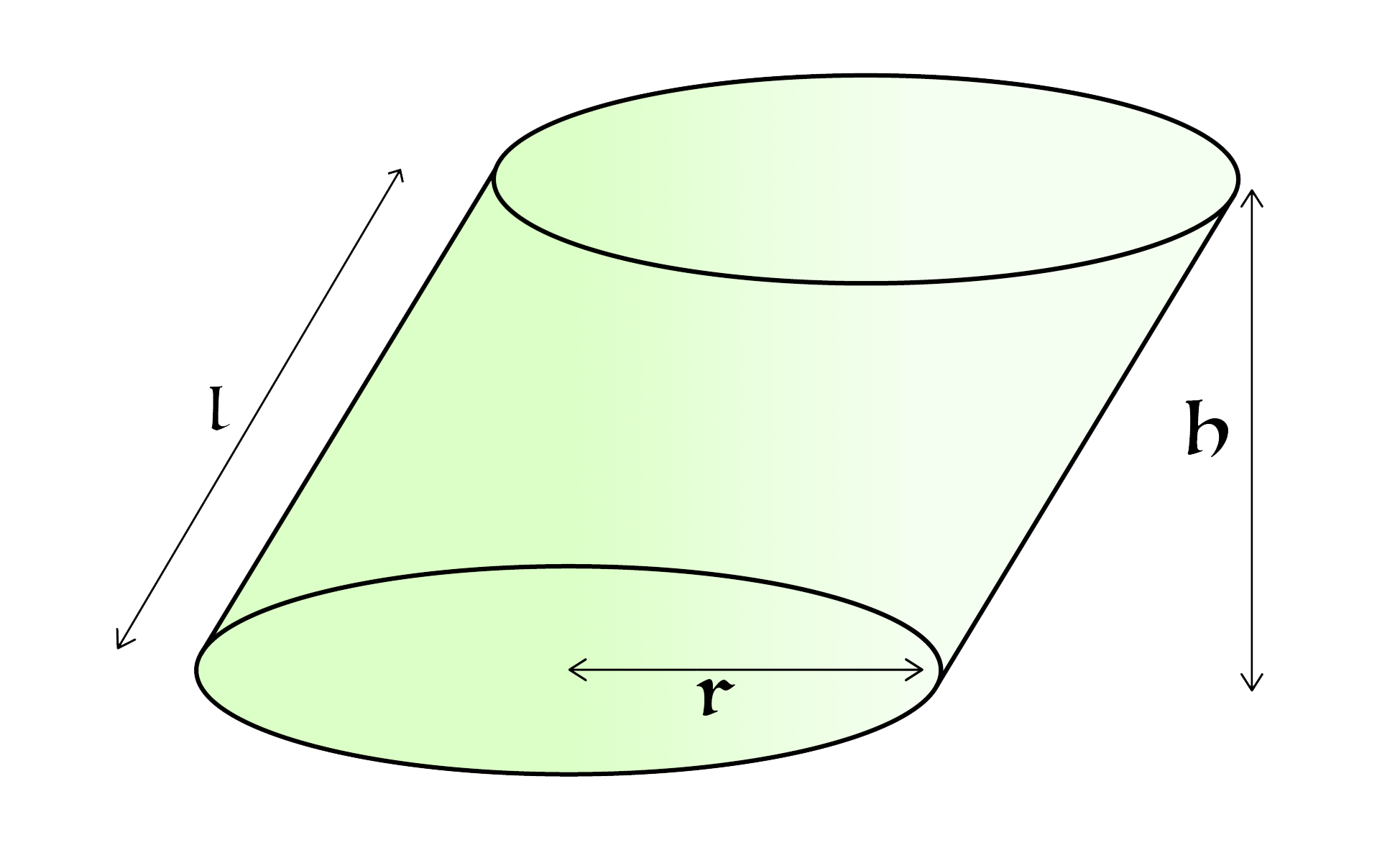Not Your Usual Cylinder - Volume

Geometry Level 3Given the following information, find the volume of the oblique cylinder shown above:

• The flat sides of the cylinder are circular, congruent and parallel to each other.
• The slant height of the cylinder is $l = 5.$
• The radius of the circular base is $r = 2 .$
• The perpendicular height of the cylinder is $h = 4.$
• The image is not drawn to scale.

State your answer to five significant figures.

×